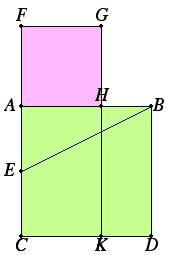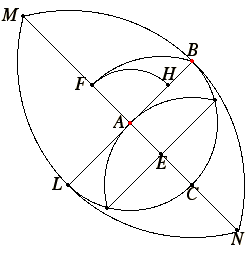# Proposition 11

To cut a given straight line so that the rectangle contained by the whole and one of the segments equals the square on the remaining segment.

Let AB be the given straight line.

It is required to cut AB so that the rectangle contained by the whole and one of the segments equals the square on the remaining segment.Describe the square ABDC on AB. Bisect AC at the point E, and join BE.

Draw CA through to F, and make EF equal to BE. Describe the square FH on AF, and draw GH through to K.

I say that AB has been cut at H so that the rectangle AB by BH equals the square on AH.

II.6

Since the straight line AC has been bisected at E, and FA is added to it, the rectangle CF by FA together with the square on AE equals the square on EF.

But EF equals EB, therefore the rectangle CF by FA together with the square on AE equals the square on EB.

I.47

But the sum of the squares on BA and AE equals the square on EB, for the angle at A is right, therefore the rectangle CF by FA together with the square on AE equals the sum of the squares on BA and AE.

Subtract the square on AE from each. Therefore the remaining rectangle CF by FA equals the square on AB.

Now the rectangle CF by FA is FK, for AF equals FG, and the square on AB is AD, therefore FK equals AD.

Subtract AK from each. Therefore FH, which remains, equals HD.

And HD is the rectangle AB by BH, for AB equals BD, and FH is the square on AH, therefore the rectangle AB by BH equals the square on HA.

Therefore the given straight line AB has been cut at H so that the rectangle AB by BH equals the square on HA.

Q.E.F.

## Guide

This construction cuts a line into two parts to solve the equation a (a – x) = x2 geometrically.

This construction is used in the proof of IV.10, which is later used to construct a regular pentagon. It accomplishes the same thing as the construction of proposition VI.30, which cuts a line into extreme and mean ratio, defined in VI.Def.3, and that construction is used later in XIII.17.

The difference between this proposition and VI.30 is a matter of terminology. Propositions dealing with ratios of lines are postponed until Book VI, but any ratio concerning lines can be converted into a statement about areas of rectangles. Proposition VI.16 states that the line A is to the line B as the line C is to the line D is equivalent to the statement that the rectangle A by D equals the rectangle B by C.

The construction of this proposition cuts a line into two parts A and B so that the rectangle A + B by A equals the square B by B. The construction in VI.30 cuts a line so that A + B : B = B : A, which by VI.16, or by its special case VI.17, is the same thing.

#### Construction steps

For the purposes of cutting the line AB, the entire diagram does not have to be constructed. The points D, G, and K are unnecessary. In the diagram to the right, only those lines and circles necessary for the construction are shown, and only those parts of them that are relevant.

Altogether, there are six circles to be drawn, two lines connected, and one line extended. In order, they are as follows. Extend BA and draw a circle centered at A with radius AB to determine the point L. Draw circles centered at B and L with radius BL to determine points M and N. Draw the straight line MN. Then C is where it meets the circle centered at A. Draw a circle centered at C with radius CA, and connect the two points where it meets the circle centered at A. Then E is where that line meets AC. Draw the circle centered at E with radius EB to determine F. Draw the circle centered at A with radius F to determine H, the desired point to cut AB.#### The golden ratio, the 36°-72°-72° triangle, and regular pentagons

This is the first of several propositions in the Elements that treats these concepts. At this point, ratios have not been introduced, so Euclid describes it in basic terms, that a given straight line is cut so that “the rectangle contained by the whole and one of the segments equals the square on the remaining segment.”